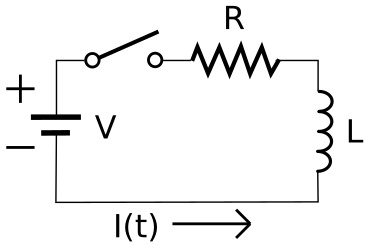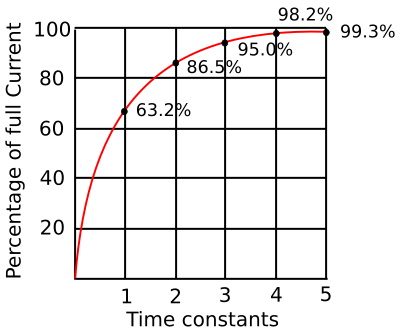#Inductors in DC Circuits by Stephen BucaroShown above is a circuit with a voltage source, a resistor, an inductor, and a switch in series. When the switch is first closed, current through the circuit is low because a magnetic field starts building in the coil, and this magnetic field creates a back electromotive force (EMF) that oppose the current in the inductor.

As the current ramps up from zero, the magnetic field builds in strength. Eventually, the current in the inductor reaches full strength, and the magnetic field stops building, so the back emf stops, and the current in the circuit reaches a steady level as calculated by the resistor and the voltage (Ohm's Law).

Note: without the back emf, the voltage across the inductor is zero, the inductor acts as a short. The entire voltage of the source is dropped across the resistor. Without the resistor in the circuit, the current would rise to the maximum capacity of the power source and the inductor would heat up and possibly burn up.The current through the inductor at any time can be calculated by the number of time constants. The length of one time constant in seconds is calculated by the formula:

T = L/R

Where

T is the time constant in seconds
L is the inductance in Henrys
R is the total circuit resistance in Ohms

Seconds and Henrys are usually too large for most calculations so milli and micro units are commonly used. Remember when calculating to convert any of these sub units to seconds or Henrys for use in formula.

In one time constant, current will have risen to 63.2% of its maximum value. After two time constants it will have reached 86.5%, after three time constants, 95% until after five time constants it reaches 99.5% which is regarded as its maximum value.

LR Series Circuit Example

Let's use the example of a circuit with a coil which has an inductance of 40mH and a resistor of 2 ohms is connected. If the source voltage is 20V DC.

t = L/R = 0.04/2 = 0.02 seconds

So five time constants would be 5t = 5 x 02 = 100 ms. After which the inductor current would be 99.5% of its maximum value.

Note, interestingly, when the switch is opened again, the resistance in the circuit becomes infinite, so a the time constant becomes an instant and the back emf is very large. This vary large voltage is across the switch, and could cause an arc, limited only by the voltage drop across the resistor.